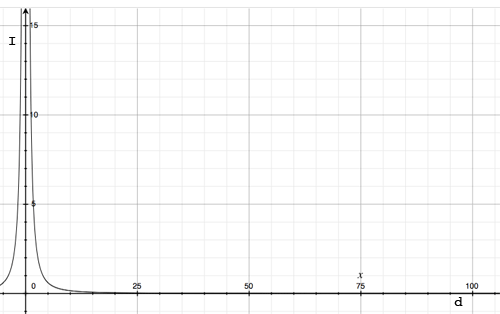SEARCH HOMEMath Central Quandaries & QueriesQuestion from Amy: Question about inverse square law ; Hi, I'm trying to understand some nuances about this law and have been reading about it a lot online and trying out some homework for personal interest (hobby ) - not school related. I understand that the simple formula is ; 1/d^2 I was wondering about what appears to be an oddity to me, that I came across when I was working with a test example I found at this link ; http://www.softschools.com/formulas/physics/inverse_square_law_formula/82/ The problem that caught my interest was this one on that page ; "1) If a bright flashlight has a light intensity of 15.0 candela at a distance 1.00 m from the lens, what is the intensity of the flashlight 100.0 m from the lens?" So I have a few different questions about this, but the most important one is, what does it mean if we replace the "1" in ; 1/d^2 with a different value such as a ratio in the form of a decimal value, so instead we have something like this ; 0.75/d^2 ??? Does this ratio represent a curve then? I am thinking that the "1" in the normal formula represents a straight line Some of the links I was researching ; http://wisptools.net/book.php?c=3&s=2 http://www.softschools.com/formulas/physics/inverse_square_law_formula/82/ https://www.nde-ed.org/GeneralResources/Formula/RTFormula/InverseSquare/InverseSquareLaw.htmHi Amy,

I want to look at the problem you quote, "If a bright flashlight has a light intensity of 15.0 candela at a distance 1.00 m from the lens, what is the intensity of the flashlight 100.0 m from the lens?" and solve it using a different approach than was used on the SoftSchools site.

They use the variable $I$ for the intensity of light in the units candela and they use the variable $d$ for the distance between the light source and the observer in meters. The statement that "The intensity of light is inversely proportional to the square of the distance." means that there is a constant $C$ so that

$I = C \times \frac{1}{d^2},$

where $C$ is in the units candela time meters squared.

The fact that $C$ is a constant means that its value is the same, regardless of the values of $I$ and $d.$

The problem also states that when $I = 15.0$ candela, $d = 1.00 m$ and hence

$15.0 = C \times \frac{1}{1.00} \mbox{ or } C = 15.0 \mbox{ candela } \times m^{2}.$

Thus we know that

$I = 15.0 \times \frac{1}{d^2}.$

The question then asks for the value of $I$ when $d = 100.0$ m. Substituting into the equation above gives

$I = 15.0 \times \frac{1}{10000} = 0.0015 \mbox{ candela.}$

To see how that looks graphically I made the horizontal axis be distance, $d$ in meters and the vertical axis intensity, $I$ in candela and plotted

$I = 15.0 \times \frac{1}{d^2}.$I hope this helps,
Penny* Registered trade mark of Imperial Oil Limited. Used under license.Math Central is supported by the University of Regina and the Imperial Oil Foundation.